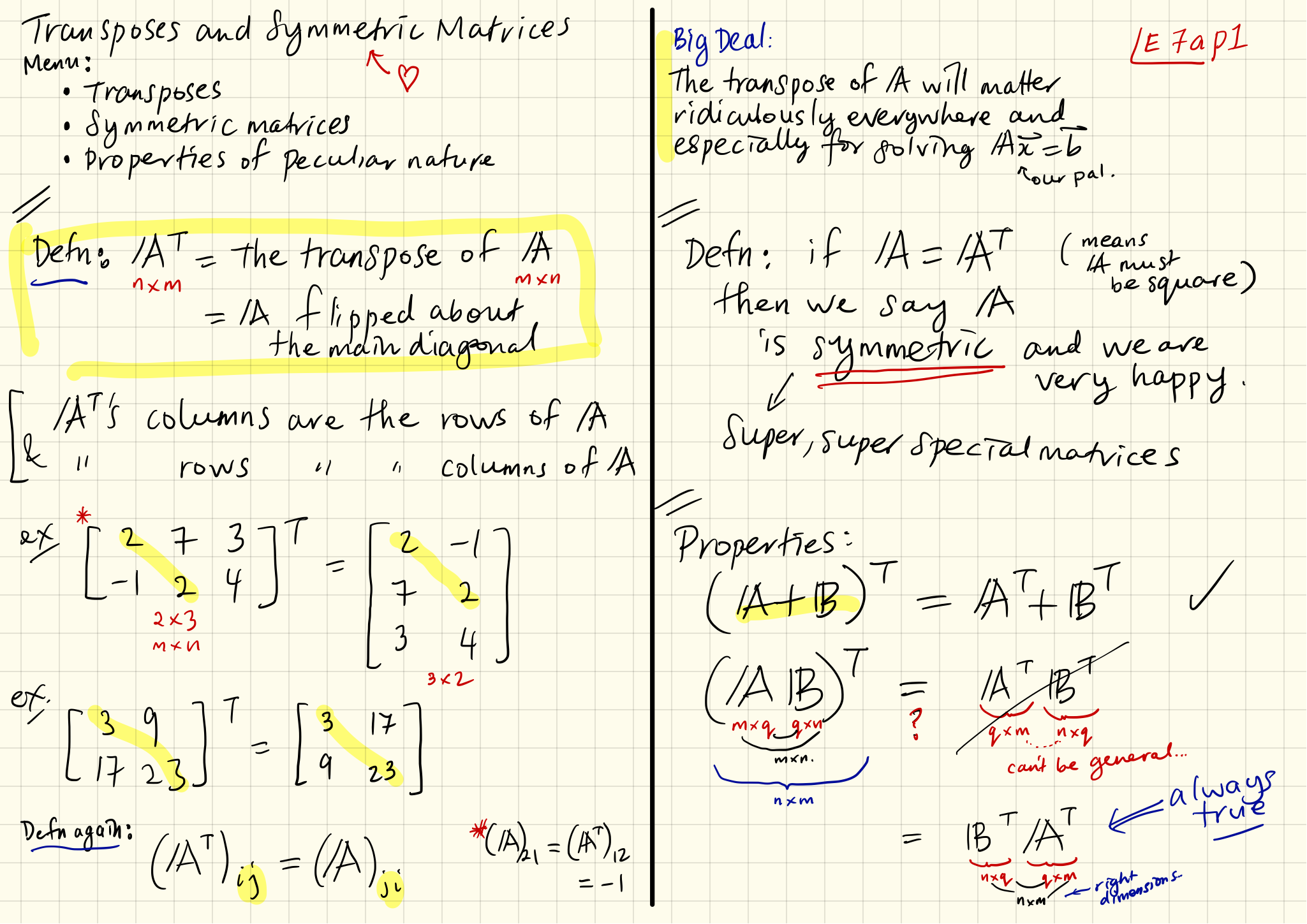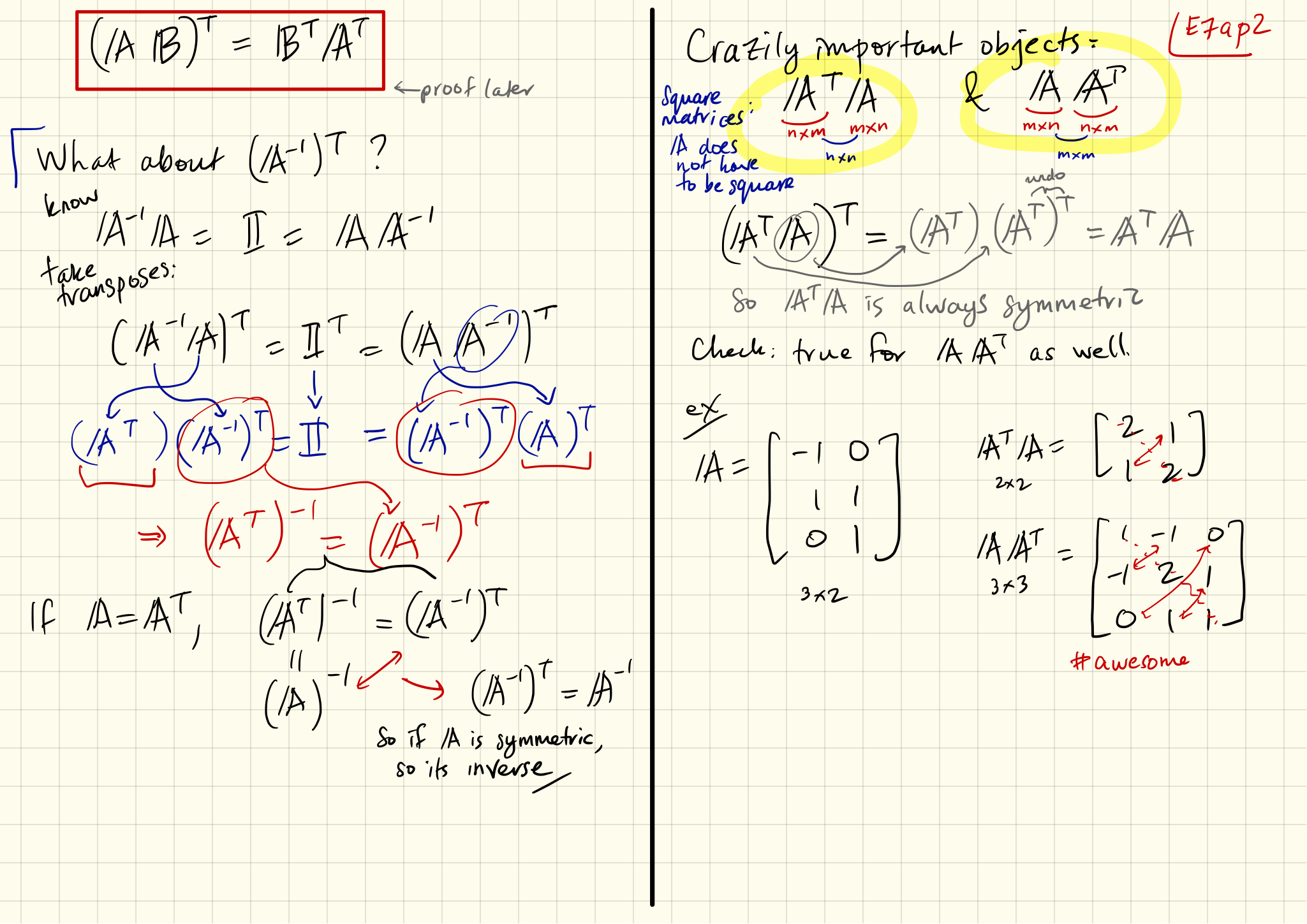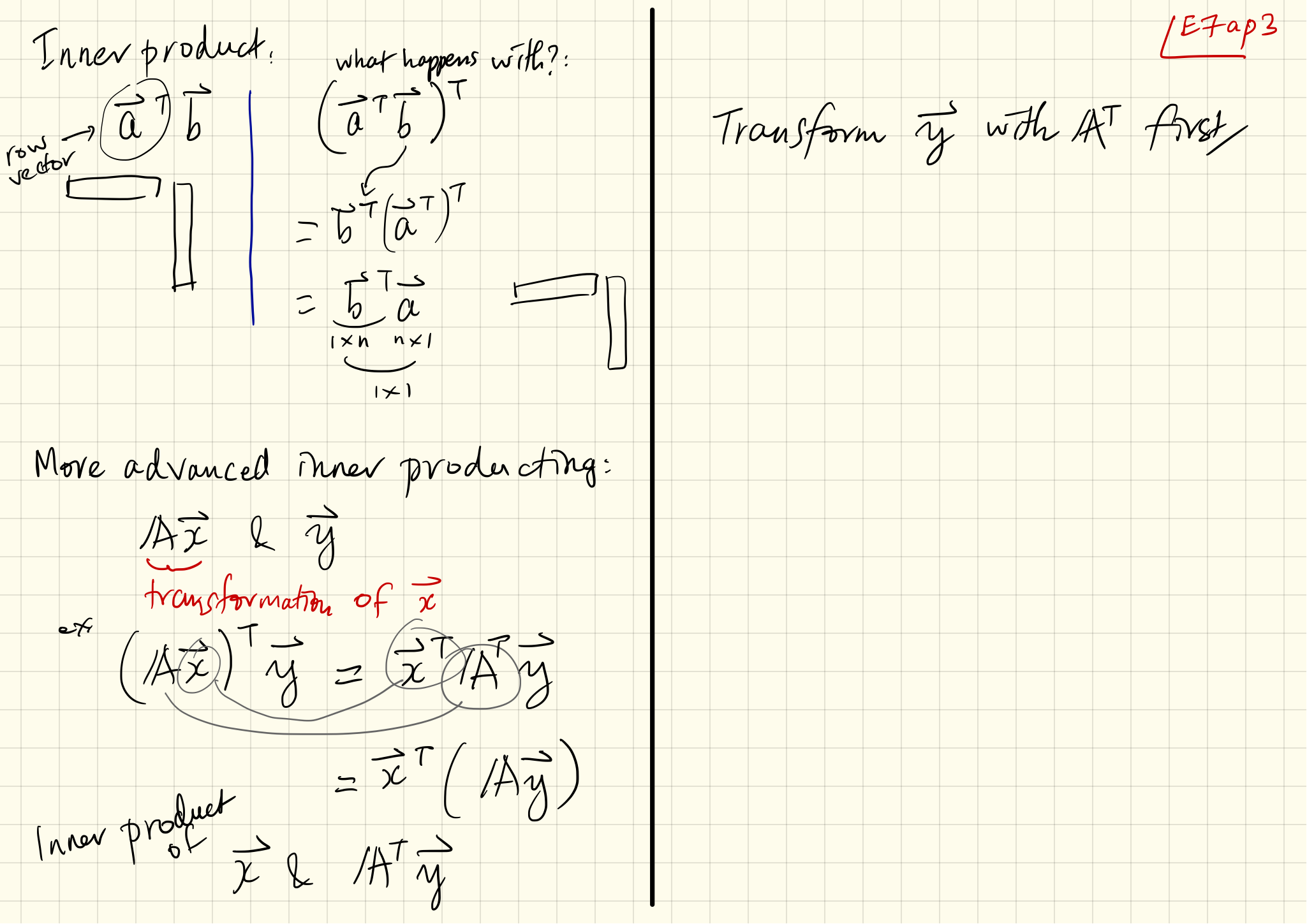Note: This is an archival, mostly functional site. All courses can be found here.

Note Set 07a: Transposes and Symmetric Matrices

We introduce the simple but amazingly essential transpose, $\mathbf{A}^{\rm T}$, the flipped version of $\mathbf{A}$ across the main diagnonal. It's a short hop to thinking about symmetric matrices, those necessarily square matrices who are the same as their transpose, $\mathbf{A} = \mathbf{A}^{\rm T}.$ We survey a number of eldritch properties of the transpose and symmetric matrices starting with the product rule $(\mathbf{AB})^{\rm T} = \mathbf{B}^{\rm T} \mathbf{A}^{\rm T}.$ Best consumed by 2016/09/16

•••